Courses

# Dynamical Systems (Part - 1) - Classical Mechanics, UGC - NET Physics Physics Notes | EduRev

## Physics : Dynamical Systems (Part - 1) - Classical Mechanics, UGC - NET Physics Physics Notes | EduRev

The document Dynamical Systems (Part - 1) - Classical Mechanics, UGC - NET Physics Physics Notes | EduRev is a part of the Physics Course Physics for IIT JAM, UGC - NET, CSIR NET.
All you need of Physics at this link: Physics
Definition

Dynamical system is a set of n first-order ordinary differential equations of the form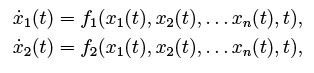(8.1)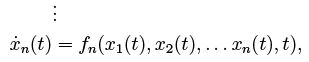where xa = xa(t) are unknown functions of time, a = 1, 2.......... n and fa are arbitrary differentiable functions of variables xa and possibly of time t. If functions fa do not depend on time explicitly, dynamical system is called autonomous, otherwise it is called non-autonomous. Using the index notation, dynamical system (8.1) can be written briefly in the form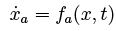(8.2)

where x stands for the n tuple of variables xa. Autonomous system is then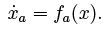In this notation we suppress time-dependence of xa on time because this dependence is assumed implicitly. Motivated by Hamilton's formalism, we intend to interpret the solution xa = xa(t) as the motion in the phase space. Phase space is an abstract space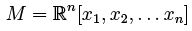with coordinates xa. Arbitrary point x ∈ M represents the state of physical system described by equations (8.1). Solution of dynamical system is not unique unless we specify the initial conditions, i.e. values of coordinates xa at some given initial time t0,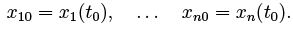Usually we set t0 = 0. The n−tuple of initial coordinates xa0 will be denoted simply by x0 ∈ M.

Suppose we choose a point x0∈ M at time t0 = 0 as in figure 8.1. A mathematical theorem guarantees that there exists unique solution x = x(t) satisfying (8.1) such that x(0) = x0. The solution x = x(t) is also called the phase trajectory. Equations (8.1) essentially state that vector f(x(t)) evaluated at arbitrary point of the trajectory is in fact tangent to the trajectory, see figure 8.1. Hence, we can interpret vector field f(x) as a velocity. Although it can be very difficult or even impossible to solve the equations of motion (8.1), the velocity gives us a good idea about the behaviour of the system.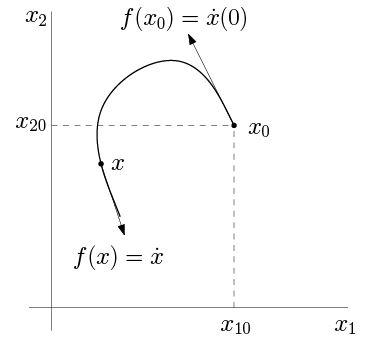Fig. 8.1. Two-dimensional dynamical system of the form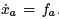. Initial position is at x0 = x(0).
The "velocity" vector at x0 is f (x0 ) and determines the tra jectory of the system in the in nitesimal neighbourhood of the initial point.

Example

Let us see an illustrating example. We are already familiar with the equation of harmonic oscillator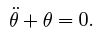This is a second order equation but we can bring into into the rs-order form by setting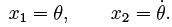Then we have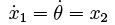and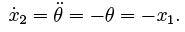Hence, instead of single equationof second order we now have two equations of first order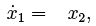(8.3)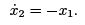Clearly, this is a dynamical system (8.1) if we set f1 = x2 and f2 = x1. Thus, the velocity eld is

f (x1; x2) = (x2; x1)                    (8.4)

This vector eld can be visualised in Mathematica by function VectorPlot: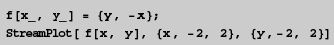Resulting gure is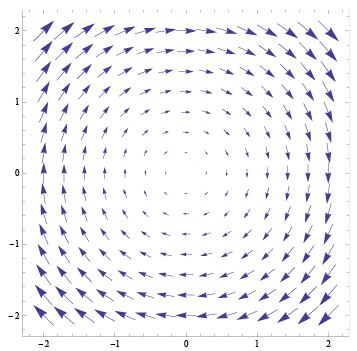This picture agrees with our previous analysis when we used the conservation of energy to show that the phase tra jectories of harmonic oscillator are circles (or ellipses when using SI units). Another possibility is to use function StreamPlot with the same arguments which yields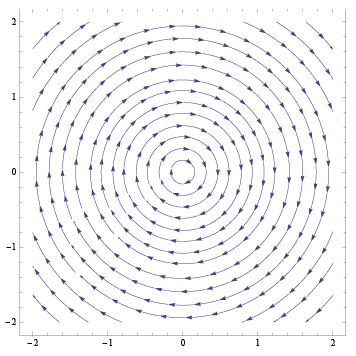Implementation in Mathematica

In this section we show how to implement a dynamical system in Mathematica in a convenient way.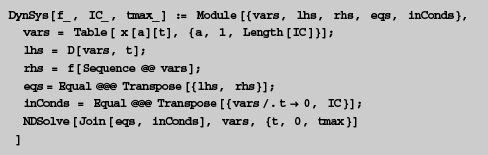This code deserves a brief explanation. Arguments of the function DynDys are

• pure function f { this is a vector function representing the right hand side of dynamical system (8.1);
• initial conditions IC { list of the initial values of variables xa at time t = 0;
• tmax { upper bound of interval t 2 (0; tmax).
Hence, function DynSys can be called, e.g. with the arguments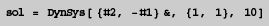In this example, pure function f is

{#2, -#1} &

which is equivalent to

f (x1; x2) = (x2; x1):

Clearly, this corresponds to harmonic oscillator (8.4).

Initial conditions IC are set to

x1(0) = 1; x2(0) = 1

and we want to nd the solution in time interval (0; 10).
Now suppose that we called function DynSys with the arguments above and let us explain how this function works. Thus, we assume that the arguments are

f = {#2, -#1}&
IC = {1, 1}
tmax = 10.

The rst command
vars = Table[ x[a][t], {a, 1, Length[IC]}];
creates a list of variables xa(t) in the form
vars = { x[t], x[t] }.
The left hand side of equations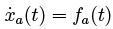is generated simply by calling

lhs = D[vars, t]

which yields

lhs = { x'[t], x'[t] }.

Now we form the right hand side of equations. Recall that vars is the list of variables. We want to evaluate functions fa at point xa, i.e. we need the expression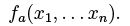However, we cannot write simply f[vars] because this would mean

f[{x[t], x[t]}]

while what we need is

f[ x[t], x[t] ].

Hence, we must turn the list vars into the sequence of arguments by replacing its head. Command

rhs = f[Sequence @@ vars].

leads to correct application of function f to arguments xa: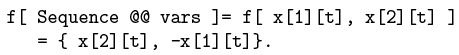Having de ned the left hand side and the right hand side of dynamical system separately, we join them in a usual way,

eqs = Equal @@@ Transpose[ {lhs, rhs} ]

Next we de ne the initial conditions with values speci ed in argument IC={1,1}. We need to produce the list

{ x == 1, x == 1 }

The left hand side of initial conditions consists of the elements of vars evaluated at time 0,

vars /. t->0,

the right hand side consists of the elements of IC. We con join them together by

inConds = Equal @@@ Transpose[{vars /. t -> 0, IC}];

Finally we solve the list of equations of motion and initial conditions by NDSolve:

NDSolve[Join[eqs, inConds], vars, {t, 0, tmax}].

Now, the reader should be familiar with functionality of function DynSys. We use this function in the following examples.

To nalize this section we show how to use function DynSys to solve the motion of harmonic oscillator.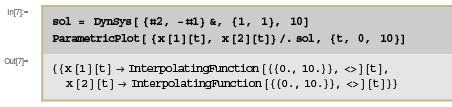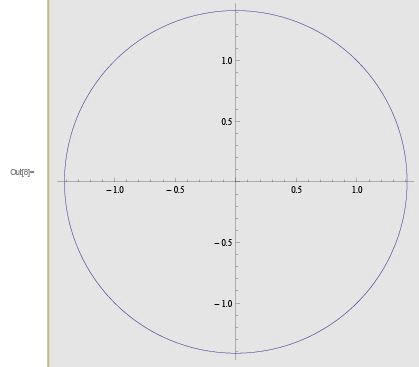Chaotic pendulum

In the previous chapters we introduced the mathematical pendulum as a simple example of physical system which has only one degree of freedom (angle of de
ection θ) and is described by the Lagrange equation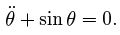This equations is non-linear because of the presence of the sine. We have seen that this equation cannot be solved in terms of elementary functions but we were able to nd the numerical solution. Moreover, using the Hamiltonian formalism we were able to plot the phase tra jectories without actually solving the equation of motion.

We can generalize the model of mathematical pendulum in several ways. First, any realistic system is dissipative, i.e. there are resisting forces acting against the motion of the pendulum. As an approximation, resisting force is proportional to velocity and has opposite direction. In the case of pendulum, velocity is proportional to θ. and hence equation of pendulum with resisting force has the form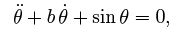where b is the constant characterizing the strength of resisting force. For example, it can be related to the viscosity of the medium in which the pendulum moves.
Pendulum with resisting force is called damped pendulum.

Next we can assume that in addition to restoring gravitational force there is an external force acting on the pendulum. Such force is called driving force. In the presence of driving force, even if the initial velocity of the pendulum is zero (and the pendulum is at equilibrium position), driving force will make the pendulum to move.
Resulting motion of the pendulum will be a "mixture" of two motions: periodic motion due to self-oscillations of the pendulum, and motion due to driving force.
Pendulum with the driving force, driven pendulum with the friction is described by equation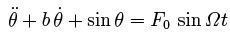(8.5)

where we assume that driving force is harmonic with angular frequency Ω and amplitude F0. In the subsequent analysis we will show that this kind of pendulum exhibits chaotic behaviour and hence we also call it chaotic pendulum.

We start with rewriting equation (8.5) in the form of dynamical system. This is straightforward since we can de ne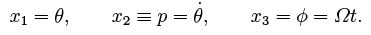We will freely pass from notation (θ, p; φ) to equivalent notation (x1, x2, x3) according to the context. By de nition, variable φ satis es equation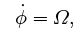while variable p (which is clearly related to the momentum of the pendulum) was de ned by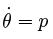which can be consequently regarded as an equation for θ. The only true dynamical equation is an equation for p which follows from (8.5):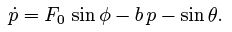Thus, variables (x1, x2, x3) = (θ, p; φ) are determined by dynamical system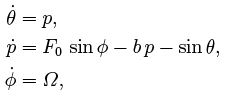(8.6)

supplemented with initial conditions, i.e. values of xa at time t = 0.

Dynamical system (8.6) can be solved in Mathematica using function DynSys de ned in the previous section. We choose initial conditions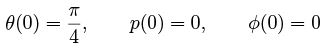and investigate how values b, Ω and F0 affect the behaviour of the pendulum. First we set

b = 0,  F0 = 0, Ω = 0.

So, we reduce driven pendulum to the case of ordinary mathematical pendulum without friction and driving force (damping coeffcient b = 0 and the amplitude of the force is F= 0).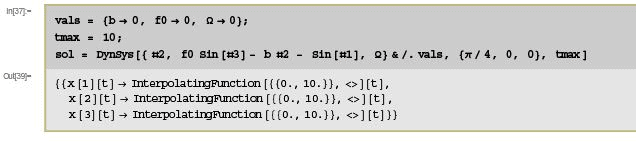Here we have chosen tmax = 10 but the reader should adjust this parameter in order to reproduce all gures below. Now we can plot the phase tra jectory in a usual way.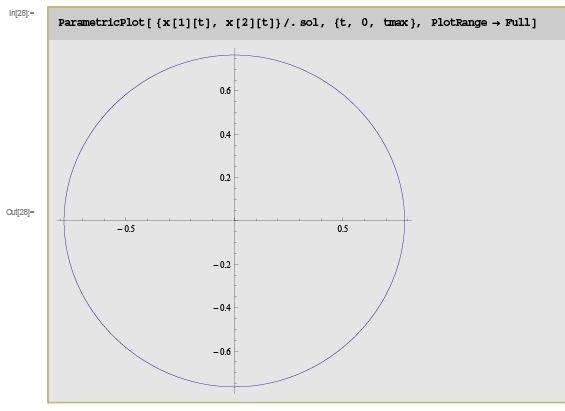Let us add the friction now and set
b = 0.1,
see gure 8.2 for the result. We can see that the phase tra jectory is a spiral which, in the limit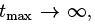ends at the origin of the phase plane. This means that the oscillations are damped until the pendulum stops. Slightly more "fancy" picture can be obtained by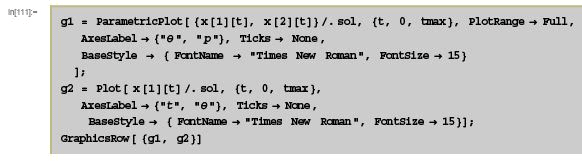which results in gure 8.3.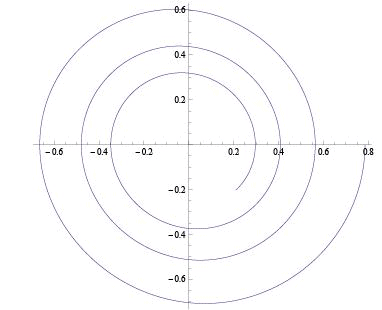Fig. 8.2. Parameters b = 0.1, F0 = 0. Non-zero friction leads to damped oscillations of the pendulum.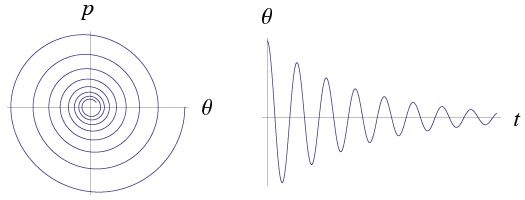Fig. 8.3. Phase tra jectory of damped pendulum together with the time dependence of deection θ = θ(t).

Let us see how the driving force affects the motion. For this purpose we set

b = 0, F= 1, Ω = 2, and choose the initial conditions to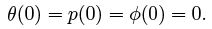In other words, the pendulum is initially at its equilibrium position and, hence, without driving force it would stay at rest. However, the presence of driving force leads to solution plotted in gure 8.4.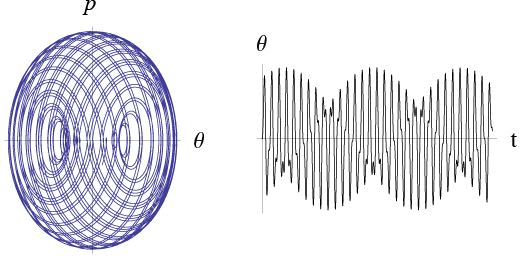Fig. 8.4. Motion of the pendulum without friction under the external driving force with amplitude F= 1 and angular frequency Ω = 2. Initial position of the pendulum is θ (0) = p(0) = 0.

In the following example we choose

b = 1, F0 = 1, Ω  = 1,

see gure 8.5. An interesting feature of this solution is the presence of short transient stage during which the phase tra jectory follows outgoing spiral but then settles at circular periodic orbit. Such behaviour is called limit cycle.

To experiment with values of parameters b, F0 and Ω and with initial values θ(0), p(0) and φ(0). We can see that resulting motion of the pendulum is a consequence of complicated and delicate interplay between three motions: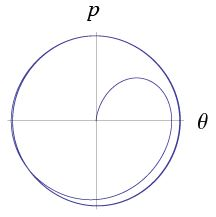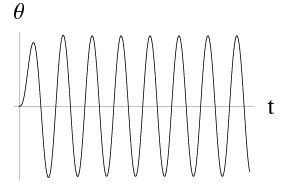Fig. 8.5. Motion of the pendulum with friction (b = 1) under the external driving force with amplitude F0 = 1 and angular frequency Ω = 1. Initial position of the pendulum is θ(0) = p(0) = 0.

•  self-oscillations of the pendulum;
• resisting force (friction);
• external driving force.

While for some combinations of parameters the motion is perfectly understandable (like in the absence of the friction and the driving force or in the absence of driving force but in the presence of friction), for general values the motion is unpredictable, chaotic.

Offer running on EduRev: Apply code STAYHOME200 to get INR 200 off on our premium plan EduRev Infinity!

159 docs

,

,

,

,

,

,

,

,

,

,

,

,

,

,

,

,

,

,

,

,

,

,

,

,

;